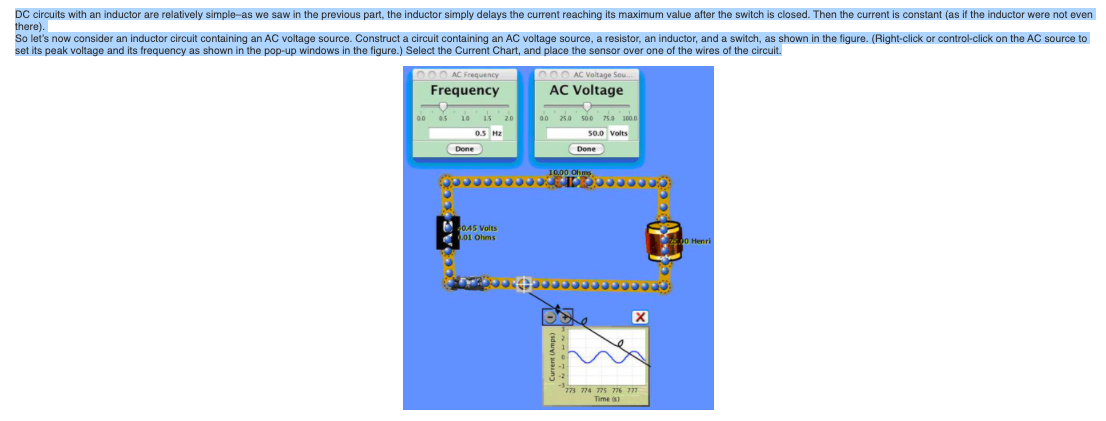# Problem: For the circuit in the previous part, with the frequency set at 1 Hz, what happens if the inductance of the inductor is increased?a. The maximum current decreases.b. The maximum current does not change.c. The maximum current increases

###### FREE Expert Solution

The inductive reactance of an inductor is given by:

$\overline{){{\mathbf{X}}}_{{\mathbf{L}}}{\mathbf{=}}{\mathbf{2}}{\mathbf{\pi }}{\mathbf{f}}{\mathbf{L}}}$

91% (430 ratings)###### Problem Details

For the circuit in the previous part, with the frequency set at 1 Hz, what happens if the inductance of the inductor is increased?

a. The maximum current decreases.

b. The maximum current does not change.

c. The maximum current increases